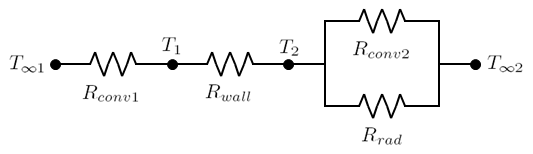f
Neutrium

# Thermal Conductivity

Thermal Conductivity of Metals and Alloys

This article provides thermal conductivity data for a selection of metals and alloys. Thermal conductivity measures a materials ability to allow heat to pass through it via conductance.

Thermal Conductivity of Common Materials

This article provides thermal conductivity data for a selection of common materials. Thermal conductivity measures a materials ability to allow heat to pass through it via conductance.

Properties of Air

This article contains formulae and tabulated data for the properties of air as a function of temperature.

Thermal ResistanceThe concept of thermal resistance can be utilised to solve steady state heat transfer problems that involve series, parallel or combined series-parallel components. This article demonstrates how to calculate the total thermal resistance for such systems and how to calculate the thermal resistance for practical geometries such as a pipe wall.

Prandtl Number

The Prandtl number is a dimensionless number named after the German physicist Ludwig Prandtl. It represents the ratio of molecular diffusivity of momentum to the molecular diffusivity of heat.